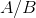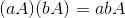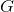# Abstract Algebra : Structure Theory

## Example Questions

### Example Question #1 : Abstract Algebra

identify the following definition.

Givenis a normal subgroup of, it is denoted thatwhen the group of left cosets ofinis called __________.

Subgroup

Cosets

Simple Group

Normal Group

Factor Group

Factor Group

Explanation:

By definition of a factor group it is stated,

Givenis a normal subgroup of, it is denoted thatwhen the group of left cosets ofinis called the factor group ofwhich is determined by.

### Example Question #4 : Abstract Algebra

Determine whether the statement is true of false:True

False

True

Explanation:

This statement is true based on the following theorem.

For allin.

Ifis a normal subgroup ofthen the cosets offorms a group under the multiplication given by,### All Abstract Algebra Resources